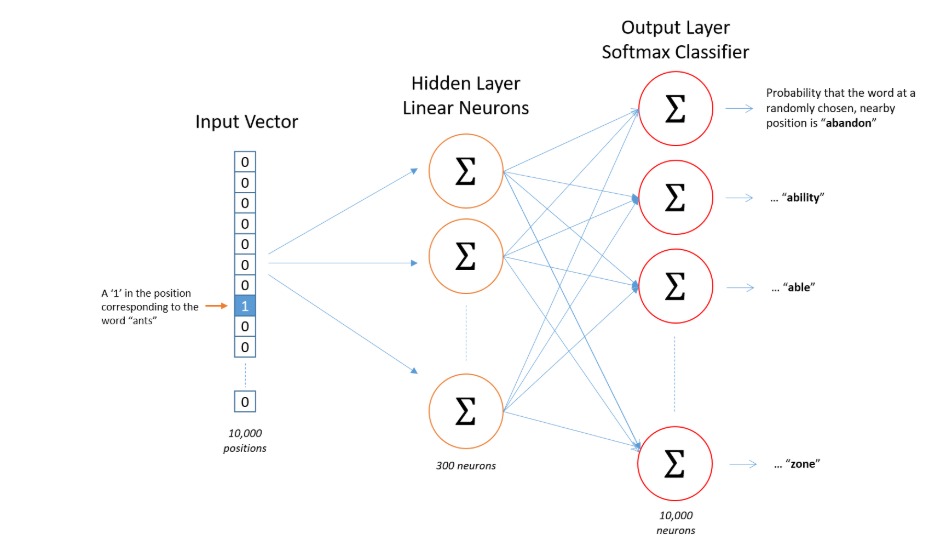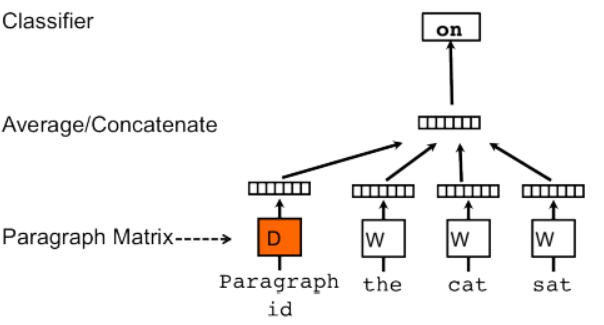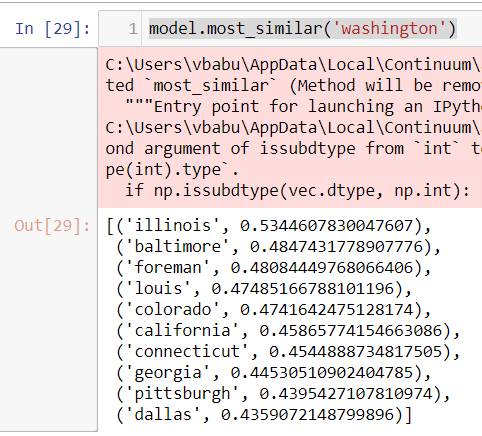# Sentence Similarity in Python using Doc2Vec

Introduction

Numeric representation of Text documents is challenging task in machine learning and there are different ways there to create the numerical features for texts such as vector representation using Bag of Words, Tf-IDF etc.I am not going in detail what are the advantages of one over the other or which is the best one to use in which case. There are lot of good reads available to explain this. My focus here is more on the doc2vec and how to use it for sentence similarity

What is Word2Vec?

It’s a Model to create the word embeddings, where it takes input as a large corpus of text and produces a vector space typically of several hundred dimesions. it was introduced in two papers between September and October 2013, by a team of researchers at Google. The underlying assumption of Word2Vec is that two words sharing similar contexts also share a similar meaning and consequently a similar vector representation from the model.

The meaning of a word can be found from the company it keeps

For instance: “Bank”, “money” and “accounts” are often used in similar situations, with similar surrounding words like “dollar”, “loan” or “credit”, and according to Word2Vec they will therefore share a similar vector representation. From this assumption, Word2Vec can be used to find out the relations between words in a dataset, compute the similarity between them, or use the vector representation of those words as input for other applications such as text classification or clustering.What is Doc2Vec?

if you understand word2vec then it would be easier to understand the Doc2vec, since it’s an extension for word2vec. So the objective of doc2vec is to create the numerical representation of sentence/paragraphs/documents unlike word2vec that computes a feature vector for every word in the corpus, Doc2Vec computes a feature vector for every document in the corpus..The vectors generated by doc2vec can be used for tasks like finding similarity between sentences/paragraphs/documentsAs per the orignal document, Paragraph Vector is capable of constructing representations of input sequences of variable length. Unlike some of the previous approaches, it is general and applicable to texts of any length: sentences, paragraphs, and documents.

Paragraph Vector framework (see Figure above), every paragraph is mapped to a unique vector, represented by a column in matrix D and every word is also mapped to a unique vector, represented by a column in matrix W. The paragraph vector and word vectors are averaged or concatenated to predict the next word in a context. In the experiments, we use concatenation as the method to combine the vectors

Check this link for Doc2vec implementation in Gensim Library

Now we will see how to use doc2vec(using Gensim) and find the Duplicate Questions pair, Competition hosted on Kaggle by Quora

Problem Statement:

Quora gets lot of duplicate questions which is added by it’s user from different locations and the main intent of Quora is to have a unique questions which can be answered by other users who are an expert or provide their opinion about the Question being asked. The primary goal of this competition is to go through the pair of questions and identify whether they are identical or not. For example, the queries “What is the most populous state in the USA?” and “Which state in the United States has the most people?” should not exist separately on Quora because the intent behind both is identical

https://www.kaggle.com/quora/question-pairs-dataset

Import Data and Cleaning

After downloading the csv file using the above Kaggle link clean the Data and drop the row if any of the questions out of the two are null Remove Stopwords using NLTK library and strip all the special characters

Check for null Questions and drop the rows

``````# Import required libraries
import pandas as pd
import pandas as pd
import numpy as np
import nltk
from nltk.corpus import stopwords
from nltk.stem import SnowballStemmer
import re
from gensim import utils
from gensim.models.doc2vec import LabeledSentence
from gensim.models.doc2vec import TaggedDocument
from gensim.models import Doc2Vec
from sklearn.metrics.pairwise import cosine_similarity
from sklearn.metrics import accuracy_score

# Import Data

# Check for null values
df[df.isnull().any(axis=1)]

# Drop rows with null Values
df.drop(df[df.isnull().any(axis=1)].index,inplace=True)
``````

Remove Stop Words

``````# Remove stop words
if remove_stopwords:
stops = set(stopwords.words("english"))
words = [w for w in text.lower().split() if not w in stops]

final_text = " ".join(words)
``````

Remove Special Characters

``````# Special Characters
review_text = re.sub(r"[^A-Za-z0-9(),!.?\'\`]", " ", final_text )
review_text = re.sub(r"\'s", " 's ", final_text )
review_text = re.sub(r"\'ve", " 've ", final_text )
review_text = re.sub(r"n\'t", " 't ", final_text )
review_text = re.sub(r"\'re", " 're ", final_text )
review_text = re.sub(r"\'d", " 'd ", final_text )
review_text = re.sub(r"\'ll", " 'll ", final_text )
review_text = re.sub(r",", " ", final_text )
review_text = re.sub(r"\.", " ", final_text )
review_text = re.sub(r"!", " ", final_text )
review_text = re.sub(r"\(", " ( ", final_text )
review_text = re.sub(r"\)", " ) ", final_text )
review_text = re.sub(r"\?", " ", final_text )
review_text = re.sub(r"\s{2,}", " ", final_text )
``````

Label all the Questions

Gensim Doc2Vec needs model training data to tag each question with a unique id, So here we would be tagging the questions with their qid using TaggedDocument API. Check the original data for the column qid1 and 1id2

Before feeding these questions to the Model, we will split each questions into different word and form list of words for each of them along with the tagging. You can see below we have used split to separate it into individual words.

``````labeled_questions=[]
labeled_questions.append(TaggedDocument(questions1[i].split(), df[df.index == i].qid1))
labeled_questions.append(TaggedDocument(questions2[i].split(), df[df.index == i].qid2))
``````

Build the Model

The labeled question is used to build the vocabulary from a sequence of sentences. This represents the vocabulary (sometimes called Dictionary in gensim) of the model. which keeps track of all unique words

``````model = Doc2Vec(dm = 1, min_count=1, window=10, size=150, sample=1e-4, negative=10)
model.build_vocab(labeled_questions)
``````

Train the Model

Model should be initialized, trained for a few epochs. This might take some time depends on your hardware configuration

``````# Train the model with 20 epochs

for epoch in range(20):
model.train(labeled_questions,epochs=model.iter,total_examples=model.corpus_count)
print("Epoch #{} is complete.".format(epoch+1))
``````

Test the Model

After the model is trained we will check if it has learnt all the words and it’s contextual meaning. We will search for “Washington” city using most_similar api and see what is the result?, It should show all the words in the document which is near or similar to Washington contextually

``````model.most_similar('washington')
``````Looks like the model is trained well and the results are coming up good here. We looked up for Washington and it gives similar Cities in US as an outputA

Cosine Similarity

We will iterate through each of the question pair and find out what is the cosine Similarity for each pair. Check this link to find out what is cosine similarity and How it is used to find similarity between two word vectors

``````score = model.n_similarity(questions1_split[i],questions2_split[i])
``````

Accuracy

There are different ways using which you can evaluate the accuracy of this model on the training data. Once the similarity score is calculated for each of the Questions pair then you can set a threshold value to find out which of the pair is duplicate or not. Since your score should be either 0 or 1 so you can set a threshold of 0.6 so if similarity score of any pair is > 0.6 then it’s a duplicate score is 1 and for any pair of question if it is <0.6 then the pair is not a duplicate and score is 0. Then pass this score to the accuracy score sklearn api with the original score in the csv file and check the accuracy of the model

``````from sklearn.metrics import accuracy_score
accuracy = accuracy_score(df.is_duplicate, scores) * 100
``````

Conclusion

We have seen that with a minimum effort how we have produce numerical features of the sentences(questions) and compare it using the cosine similarity to find out whether the question pair is duplicate or not. Additionaly, As a next step you can use the Bag of Words or TF-IDF model to covert these texts into numerical feature and check the accuracy score using cosine similarity.

To conclude - if you have a document related task then DOC2Vec is the ultimate way to convert the documents into numerical vectors.

Tags:

Categories:

Updated: